# MATLAB. Design your own low-cut shelving filter which can cut the low frequency of given music signal. Include magnitude...

MATLAB. Design your own low-cut shelving filter which can cut the low frequency of given music signal. Include magnitude and phase plot of your filter.

```rng default

Fs = 1000;
t = linspace(0,1,Fs);
x = cos(2*pi*100*t)+0.5*randn(size(t));```

The filter design is an FIR lowpass filter with order equal to 20 and a cutoff frequency of 150 Hz. Use a Kaiser window with length one sample greater than the filter order and β=3. See kaiser for details on the Kaiser window.

Use fir1 to design the filter. fir1 requires normalized frequencies in the interval [0,1], where 1 corresponds to π rad/sample. To use fir1, you must convert all frequency specifications to normalized frequencies.

Design the filter and view the filter's magnitude response.

```fc = 150;
Wn = (2/Fs)*fc;
b = fir1(20,Wn,'low',kaiser(21,3));

fvtool(b,1,'Fs',Fs)```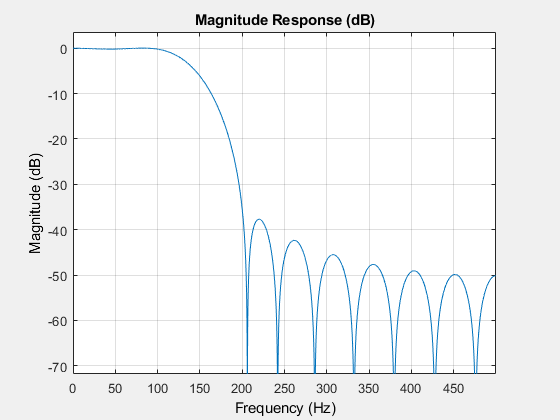Apply the filter to the signal and plot the result for the first ten periods of the 100 Hz sinusoid.

```y = filter(b,1,x);

plot(t,x,t,y)
xlim([0 0.1])

xlabel('Time (s)')
ylabel('Amplitude')
legend('Original Signal','Filtered Data')```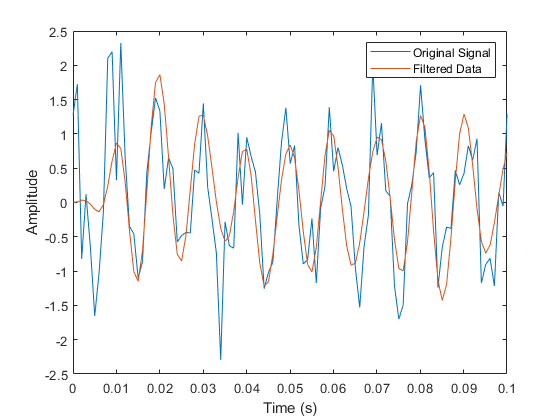Design the same filter using designfilt. Set the filter response to 'lowpassfir' and input the specifications as Name,Value pairs. With designfilt, you can specify your filter design in Hz.

```Fs = 1000;
Hd = designfilt('lowpassfir','FilterOrder',20,'CutoffFrequency',150, ...
'DesignMethod','window','Window',{@kaiser,3},'SampleRate',Fs);```

Filter the data and plot the result.

```y1 = filter(Hd,x);

plot(t,x,t,y1)
xlim([0 0.1])

xlabel('Time (s)')
ylabel('Amplitude')
legend('Original Signal','Filtered Data')```Lowpass FIR Filter with Filter Designer

This example shows how to design and implement a lowpass FIR filter using the window method with the interactive Filter Designer app.

• Start the app by entering filterDesigner at the command line.

• Set the Response Type to Lowpass.

• Set the Design Method to FIR and select the Window method.

• Under Filter Order, select Specify order. Set the order to 20.

• Under Frequency Specifications, set Units to Hz, Fs to 1000, and Fc to 150.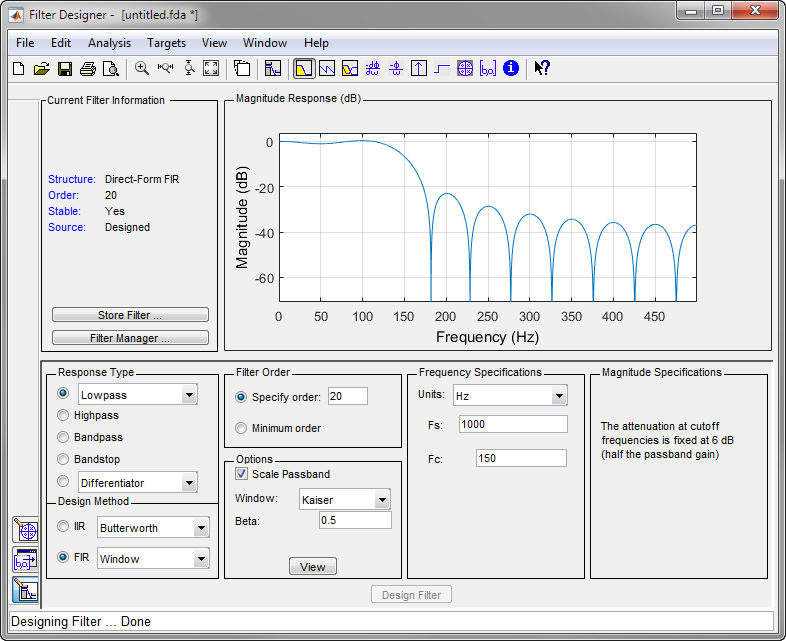• Click Design Filter.

• Select File > Export... to export your FIR filter to the MATLAB® workspace as coefficients or a filter object. In this example, export the filter as an object. Specify the variable name as Hd.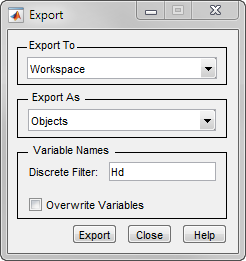• Click Export.

• Filter the input signal in the command window with the exported filter object. Plot the result for the first ten periods of the 100 Hz sinusoid.

```y2 = filter(Hd,x);

plot(t,x,t,y2)
xlim([0 0.1])

xlabel('Time (s)')
ylabel('Amplitude')
legend('Original Signal','Filtered Data')```• Select File > Generate MATLAB Code > Filter Design Function to generate a MATLAB function to create a filter object using your specifications.

You can also use the interactive tool filterBuilder to design your filter.

Bandpass Filters – Minimum-Order FIR and IIR Systems

This example shows how to design a bandpass filter and filter data with minimum-order FIR equiripple and IIR Butterworth filters. You can model many real-world signals as a superposition of oscillating components, a low-frequency trend, and additive noise. For example, economic data often contain oscillations, which represent cycles superimposed on a slowly varying upward or downward trend. In addition, there is an additive noise component, which is a combination of measurement error and the inherent random fluctuations in the process.

In these examples, assume you sample some process every day for one year. Assume the process has oscillations on approximately one-week and one-month scales. In addition, there is a low-frequency upward trend in the data and additive N(0,1/4) white Gaussian noise.

Create the signal as a superposition of two sine waves with frequencies of 1/7 and 1/30 cycles/day. Add a low-frequency increasing trend term and N(0,1/4) white Gaussian noise. Reset the random number generator for reproducible results. The data is sampled at 1 sample/day. Plot the resulting signal and the power spectral density (PSD) estimate.

```rng default

Fs = 1;
n = 1:365;

x = cos(2*pi*(1/7)*n)+cos(2*pi*(1/30)*n-pi/4);
trend = 3*sin(2*pi*(1/1480)*n);

y = x+trend+0.5*randn(size(n));

[pxx,f] = periodogram(y,[],[],Fs);

subplot(2,1,1)
plot(n,y)
xlim([1 365])
xlabel('Days')
grid

subplot(2,1,2)
plot(f,10*log10(pxx))
xlabel('Cycles/day')
ylabel('dB')
grid```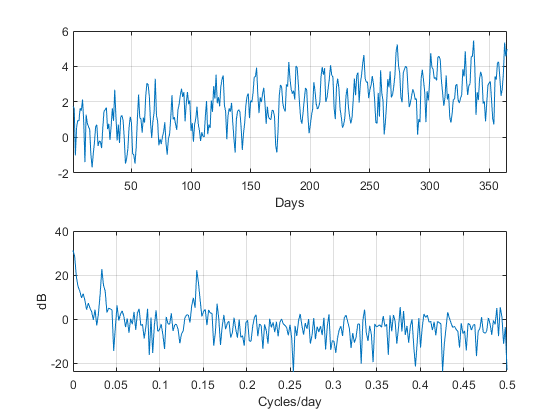The low-frequency trend appears in the power spectral density estimate as increased low-frequency power. The low-frequency power appears approximately 10 dB above the oscillation at 1/30 cycles/day. Use this information in the specifications for the filter stopbands.

Design minimum-order FIR equiripple and IIR Butterworth filters with the following specifications: passband from [1/40,1/4] cycles/day and stopbands from [0,1/60] and [1/4,1/2] cycles/day. Set both stopband attenuations to 10 dB and the passband ripple tolerance to 1 dB.

```Hd1 = designfilt('bandpassfir', ...
'StopbandFrequency1',1/60,'PassbandFrequency1',1/40, ...
'PassbandFrequency2',1/4 ,'StopbandFrequency2',1/2 , ...
'StopbandAttenuation1',10,'PassbandRipple',1, ...
'StopbandAttenuation2',10,'DesignMethod','equiripple','SampleRate',Fs);
Hd2 = designfilt('bandpassiir', ...
'StopbandFrequency1',1/60,'PassbandFrequency1',1/40, ...
'PassbandFrequency2',1/4 ,'StopbandFrequency2',1/2 , ...
'StopbandAttenuation1',10,'PassbandRipple',1, ...
'StopbandAttenuation2',10,'DesignMethod','butter','SampleRate',Fs);```

Compare the order of the FIR and IIR filters and the unwrapped phase responses.

`fprintf('The order of the FIR filter is %d\n',filtord(Hd1))`
```The order of the FIR filter is 78
```
`fprintf('The order of the IIR filter is %d\n',filtord(Hd2))`
```The order of the IIR filter is 8
```
```[phifir,w] = phasez(Hd1,[],1);
[phiiir,w] = phasez(Hd2,[],1);

figure
plot(w,unwrap(phifir))
hold on
plot(w,unwrap(phiiir))
hold off

xlabel('Cycles/Day')
legend('FIR Equiripple Filter','IIR Butterworth Filter')
grid```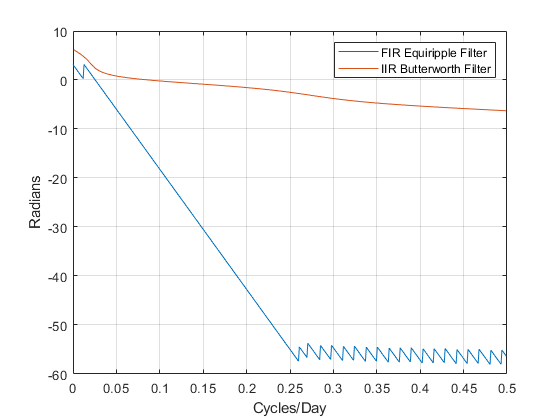The IIR filter has a much lower order that the FIR filter. However, the FIR filter has a linear phase response over the passband, while the IIR filter does not. The FIR filter delays all frequencies in the filter passband equally, while the IIR filter does not.

Additionally, the rate of change of the phase per unit of frequency is greater in the FIR filter than in the IIR filter.

Design a lowpass FIR equiripple filter for comparison. The lowpass filter specifications are: passband [0,1/4] cycles/day, stopband attenuation equal to 10 dB, and the passband ripple tolerance set to 1 dB.

```Hdlow = designfilt('lowpassfir', ...
'PassbandFrequency',1/4,'StopbandFrequency',1/2, ...
'PassbandRipple',1,'StopbandAttenuation',10, ...
'DesignMethod','equiripple','SampleRate',1);```

Filter the data with the bandpass and lowpass filters.

```yfir = filter(Hd1,y);
yiir = filter(Hd2,y);
ylow = filter(Hdlow,y);```

Plot the PSD estimate of the bandpass IIR filter output. You can replace yiir with yfir in the following code to view the PSD estimate of the FIR bandpass filter output.

```[pxx,f] = periodogram(yiir,[],[],Fs);

plot(f,10*log10(pxx))

xlabel('Cycles/day')
ylabel('dB')
grid```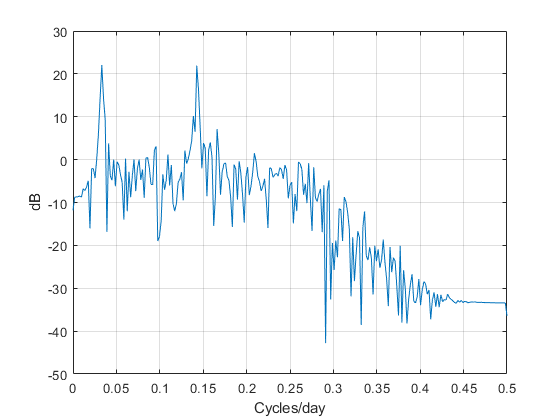The PSD estimate shows the bandpass filter attenuates the low-frequency trend and high-frequency noise.

Plot the first 120 days of FIR and IIR filter output.

```plot(n,yfir,n,yiir)

axis([1 120 -2.8 2.8])
xlabel('Days')
legend('FIR bandpass filter output','IIR bandpass filter output', ...
'Location','SouthEast')```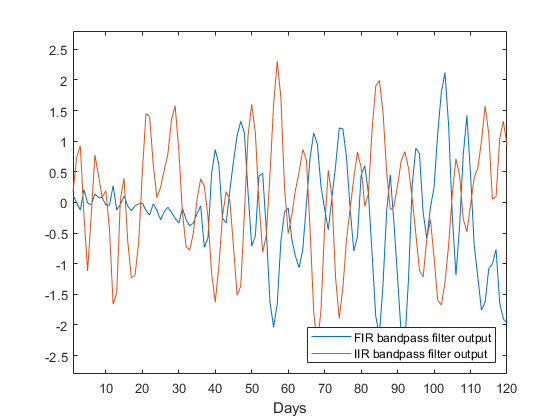The increased phase delay in the FIR filter is evident in the filter output.

Plot the lowpass FIR filter output superimposed on the superposition of the 7-day and 30-day cycles for comparison.

```plot(n,x,n,ylow)

xlim([1 365])
xlabel('Days')
legend('7-day and 30-day cycles','FIR lowpass filter output', ...
'Location','NorthWest')```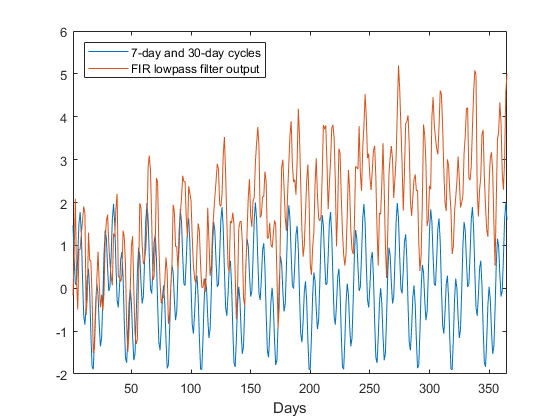You can see in the preceding plot that the low-frequency trend is evident in the lowpass filter output. While the lowpass filter preserves the 7-day and 30-day cycles, the bandpass filters perform better in this example because the bandpass filters also remove the low-frequency trend.

Zero-Phase Filtering

This example shows how to perform zero-phase filtering.

Repeat the signal generation and lowpass filter design with fir1 and designfilt. You do not have to execute the following code if you already have these variables in your workspace.

```rng default

Fs = 1000;
t = linspace(0,1,Fs);
x = cos(2*pi*100*t)+0.5*randn(size(t));

% Using fir1
fc = 150;
Wn = (2/Fs)*fc;
b = fir1(20,Wn,'low',kaiser(21,3));

% Using designfilt
Hd = designfilt('lowpassfir','FilterOrder',20,'CutoffFrequency',150, ...
'DesignMethod','window','Window',{@kaiser,3},'SampleRate',Fs);```

Filter the data using filter. Plot the first 100 points of the filter output along with a superimposed sinusoid with the same amplitude and initial phase as the input signal.

```yout = filter(Hd,x);
xin = cos(2*pi*100*t);

plot(t,xin,t,yout)
xlim([0 0.1])

xlabel('Time (s)')
ylabel('Amplitude')
legend('Input Sine Wave','Filtered Data')
grid```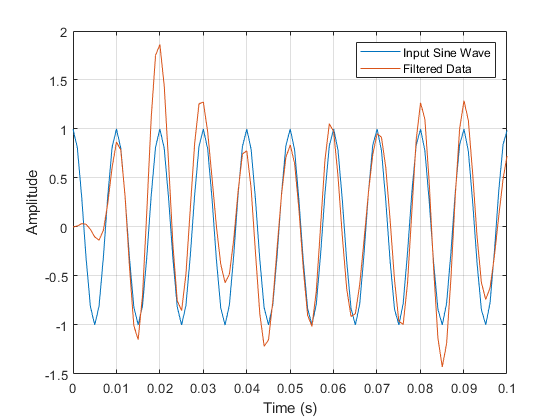Looking at the initial 0.01 seconds of the filtered data, you see that the output is delayed with respect to the input. The delay appears to be approximately 0.01 seconds, which is almost 1/2 the length of the FIR filter in samples (10×0.001).

This delay is due to the filter's phase response. The FIR filter in these examples is a type I linear-phase filter. The group delay of the filter is 10 samples.

Plot the group delay using fvtool.

`fvtool(Hd,'Analysis','grpdelay')`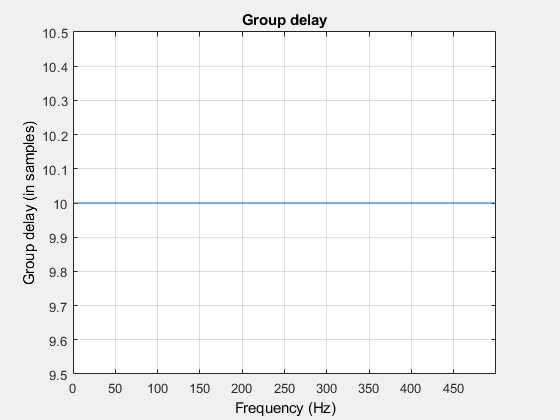In many applications, phase distortion is acceptable. This is particularly true when phase response is linear. In other applications, it is desirable to have a filter with a zero-phase response. A zero-phase response is not technically possibly in a noncausal filter. However, you can implement zero-phase filtering using a causal filter with filtfilt.

Filter the input signal using filtfilt. Plot the responses to compare the filter outputs obtained with filter and filtfilt.

```yzp = filtfilt(Hd,x);

plot(t,xin,t,yout,t,yzp)

xlim([0 0.1])
xlabel('Time (s)')
ylabel('Amplitude')
legend('100-Hz Sine Wave','Filtered Signal','Zero-phase Filtering',...
'Location','NorthEast')```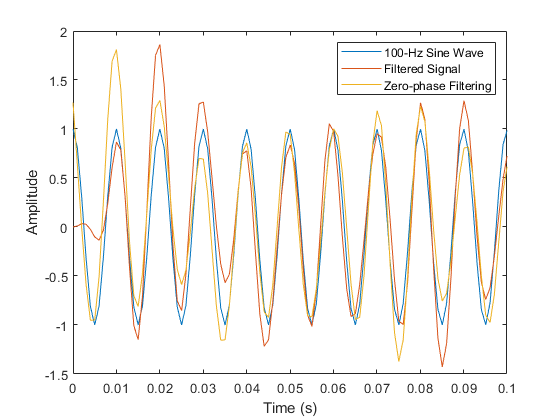##### Add Answer of: MATLAB. Design your own low-cut shelving filter which can cut the low frequency of given music signal. Include magnitude...
Similar Homework Help Questions
• ### MATLAB. Design your own low-pass shelving filter which can boost the low frequency of given music signal. After designin...

MATLAB. Design your own low-pass shelving filter which can boost the low frequency of given music signal. After designing filter, apply the filter to the original music signal and observe the result. Include following plots. A. Magnitude and phase plot of your filter. B. Magnitude plot of original signal C. Magnitude plot of filtered signal

• ### MATLAB. Design your own low-pass shelving filter which can boost the low frequency of given music signal. After designin...

MATLAB. Design your own low-pass shelving filter which can boost the low frequency of given music signal. After designing filter, apply the filter to the original music signal and observe the result. Include following plots. A. Magnitude and phase plot of your filter. B. Magnitude plot of original signal C. Magnitude plot of filtered signal

• ### Below is the MATLAB code of low-cut shelving filter which can cut the low frequency of given music signal and low-boost...

Below is the MATLAB code of low-cut shelving filter which can cut the low frequency of given music signal and low-boost shelving filter which can boost the low frequency of given music signal. Design your low-boost shelving filter and low-cut shelving filter to have noticeablly different sound. Compare the sounds of two music signals after filtering, and explain the difference in sounds briefly. If there are any mistakes in code, correct them.   Low-cut shelving filter code: close all, clear all,...

• ### 5) Design a one-pole, one-zero passive filter to have a low-frequency gain of -32 dB, a high-freq...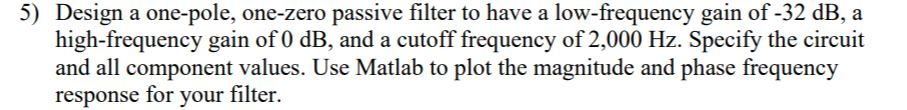please show all steps and matlab plot , 5) Design a one-pole, one-zero passive filter to have a low-frequency gain of -32 dB, a high-frequency gain of 0 dB, and a cutoff frequency of 2,000 Hz. Specify the circuit and all component values. Use Matlab to plot the magnitude and phase frequency response for your filter. 5) Design a one-pole, one-zero passive filter to have a low-frequency gain of -32 dB, a high-frequency gain of 0 dB, and a cutoff...

• ### Using filterDesigner in MATLAB, design a second order low pass IIR Butterworth filter whose sampling frequency (Fs) is 1...

Using filterDesigner in MATLAB, design a second order low pass IIR Butterworth filter whose sampling frequency (Fs) is 1 kHz and cutoff frequency (Fc) is 10 Hz. Find the numerator and denominator coefficients. Write its transfer function H(z) = Y(z) / X(z). Write its difference function y(k). Draw (copy from Filter Designer) the magnitude response plot. Draw (copy from Filter Designer) the phase response plot. Draw (copy from Filter Designer) the impulse response plot.

• ### A) Design a low-pass filter using the given circuitry with a cut-off value of 1 kHz and plot the ...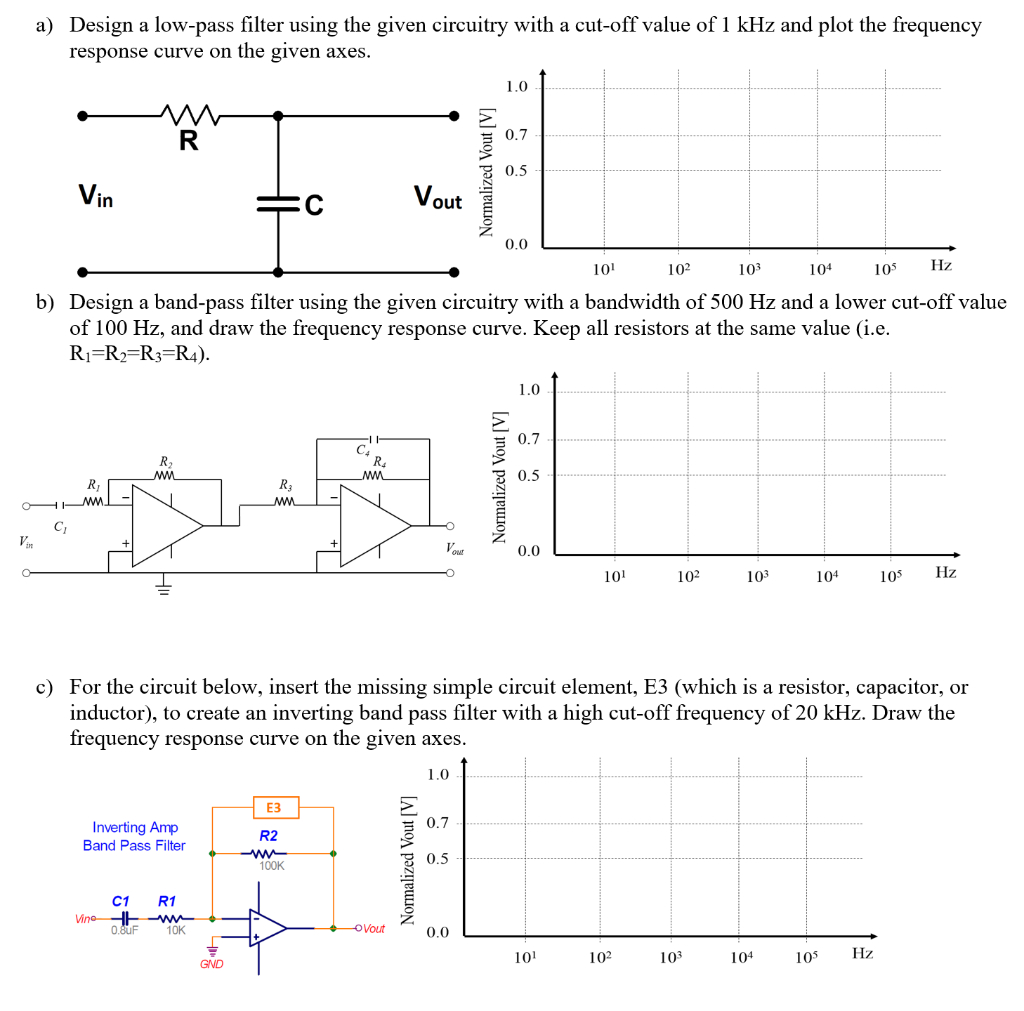a) Design a low-pass filter using the given circuitry with a cut-off value of 1 kHz and plot the frequency response curve on the given axes 1.0 0.7 0.5 in out 0.0 101 102 103 104 10s Hz b) Design a band-pass filter using the given circuitry with a bandwidth of 500 Hz and a lower cut-off value of 100 Hz, and draw the frequency response curve. Keep all resistors at the same value (i.e. Ri-R-R3-R4). 1.0 0.7 0.5 0.0...

• ### Design lowpass IIR filter with the following specifications: Filter order = 2, Butterworth type C...

Design lowpass IIR filter with the following specifications: Filter order = 2, Butterworth type Cut-off frequency=800 Hz Sampling rate =8000 Hz Design using the bilinear z-transform design method Print the lowpass IIR filter coefficients and plot the frequency responses using MATLAB. MATLAB>>freqz(bLP,aLP,512,8000); axis([0 4000 –40 1]); Label and print your graph. What is the filter gain at the cut-off frequency 800 Hz? What are the filter gains for the stopband at 2000 Hz and the passband at 50 Hz based...

• ### Problem 4: Design a first-order, strictly causal, low-pass DT filter to recover a low frequency sensor signal, corr...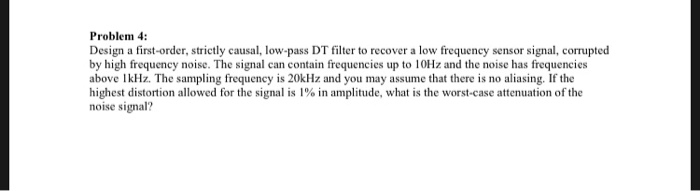Problem 4: Design a first-order, strictly causal, low-pass DT filter to recover a low frequency sensor signal, corrupted by high frequency noise. The signal can contain frequencies up to 10HZ and the noise has frequencies above IkHz. The sampling frequency is 20kHz and you may assume that there is no aliasing. If the highest distortion allowed for the signal is 1% in amplitude, what is the worst-case attenuation of the noise signal? Problem 4: Design a first-order, strictly causal, low-pass...

• ### Using the windowing functions discussed in class, design a low-pass FIR filter with a cutoff freq...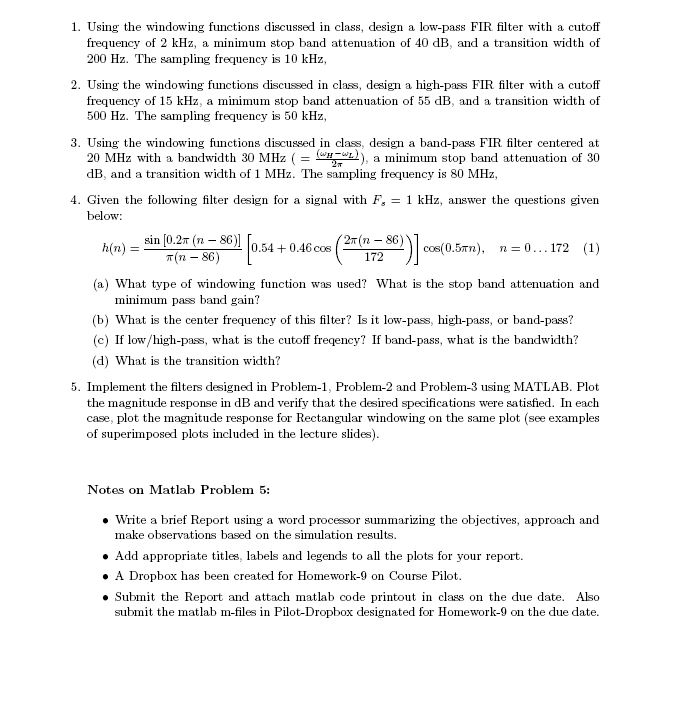Using the windowing functions discussed in class, design a low-pass FIR filter with a cutoff frequency of 2 kHz, a minimum stop band attenuation of 40 dB, and a transition width of 200Hz. The sampling frequency is 10kHz. 1. Using the windowing functions discussed in class, design a low-pass FIR filter with a cutoff frequency of 2 kHz, a minimum stop band attenuation of 40 dB, and a transition width of 200 Hz. The sampling frequency is 10 kHz 2....

• ### DSP low pass filter design simple problem using MATLAB

This homework is due Tusday, and i have no clue using matlab and the professor didn't explain much ... can anybody help please ???Design a lowpass FIR filter with the following specifications using MATLAB:Number of filter taps = determined on your trialsSampling rate =8000 HzPassband: 0 - 1200 HzStopband 1500 - 4000 HzPassband ripple: 1 dBStopband attenuation: 40 dB(a) Print the lowpass FIR filter coefficients and plot the frequency responses using MATLAB. Label and print your graph.(b) Determine the ripples...

Need Online Homework Help?